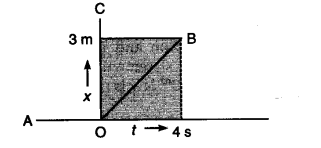# The given figure shows the position time graph of a particle of mass 4 kg

The given figure shows the position time graph of a particle of mass 4 kg. What is the force on the particle for (i) t < 0, (ii) 0 < t < 4 s, and (iii) f > 4s? The motion may be considered as one dimensional.(i) AO is along horizontal axis, i.e., distance moved is nil so force on particle is zero.
(ii) OB shows uniform velocity so force on particle is zero.
(iii) BC shows same distance all the time i.e., the body is at rest so force on the particle is zero.